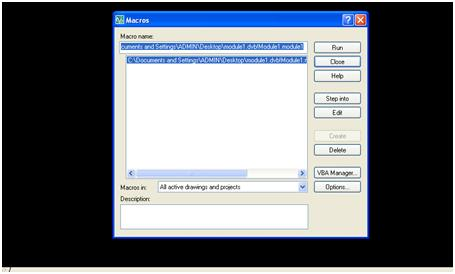# Computer Aided Automation

Full Version: Example 1 : Draw a line using AutoCAD VBA
You're currently viewing a stripped down version of our content. View the full version with proper formatting.
Example 1 : Draw a line using AutoCAD VBA

'Set Start point and End Point Variables
Dim Startpoint(0 To 2) As Double
Dim Endpoint(0 To 2) As Double
'Start Coordinates XYZ values
Startpoint(0) = 100: Startpoint(1) = 100: Startpoint(2) = 0
'End Coordinates XYZ values
Endpoint(0) = 200: Endpoint(1) = 200: Endpoint(2) = 0
'Line object in to drawing space

This programs uses 2 variables called Startpoint and Endoint to store the values of X,Y,Z coordinates of start point and end point of the line to be drawn, X1, Y1, and Z1 belongs to Startpoint where as X2,Y2 and Z2 belongs to Endpoint.
In this example, X1 = 100; Y1 = 100 and Z1 = 0 (z value is 0 for 2D drawings) and X2 = 200; Y2 = 100 and Z2 = 0.
Just copy and paste the above program snippet in between, Sub module1 () and End Sub
And final code will be something like below mentioned

Sub module1()
On Error Resume Next        ‘ To bypass run time error occurred (if any)
'Set Start point and End Point Variables
Dim Startpoint(0 To 2) As Double
Dim Endpoint(0 To 2) As Double
'Start Coordinates XYZ values
Startpoint(0) = 100: Startpoint(1) = 100: Startpoint(2) = 0
'End Coordinates XYZ values
Endpoint(0) = 200: Endpoint(1) = 200: Endpoint(2) = 0
'Line object in to drawing space